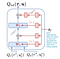# QMIX paper ripped: Monotonic Value Function Factorization for Deep Multi-agent Reinforcement Learning in StarCraft II

`import torch.nn as nnimport torch.nn.functional as Fclass RNNAgent(nn.Module):    def __init__(self, input_shape, args):        super(RNNAgent, self).__init__()        self.args = args        self.fc1 = nn.Linear(input_shape, args.rnn_hidden_dim)        self.rnn = nn.GRUCell(args.rnn_hidden_dim, args.rnn_hidden_dim)        self.fc2 = nn.Linear(args.rnn_hidden_dim, args.n_actions)def init_hidden(self):        # make hidden states on same device as model        return self.fc1.weight.new(1, self.args.rnn_hidden_dim).zero_()`Hypernetwork that calculates the forced positive weights of the Mixing Network
`class QMixer(nn.Module):    def __init__(self, args):        super(QMixer, self).__init__()         self.args = args        self.n_agents = args.n_agents        self.state_dim = int(np.prod(args.state_shape))         self.embed_dim = args.mixing_embed_dim         self.hyper_w_1 = nn.Linear(self.state_dim, self.embed_dim * self.n_agents)        self.hyper_w_final = nn.Linear(self.state_dim, self.embed_dim)         # State dependent bias for hidden layer        self.hyper_b_1 = nn.Linear(self.state_dim, self.embed_dim)# V(s) instead of a bias for the last layers        self.V = nn.Sequential(nn.Linear(self.state_dim, self.embed_dim),                               nn.ReLU(),                               nn.Linear(self.embed_dim, 1))`

Written by